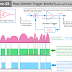A Schmitt Trigger is basically a comparator circuit that is used to convert an analog signal into a digital signal. The main difference between an ordinary comparator and a Schmitt trigger is that the output of the ordinary comparator is badly affected by the noise elements in the input signal whereas the output of a Schmitt trigger does not affect by the noise elements of the input signal, so it provides a clean and efficient output. So the main function of the Schmitt trigger is to convert the analog signal into a digital signal with minimum noise.

The Schmitt trigger circuit is built by applying the positive feedback to the noninverting input of a comparator or differential amplifier.

The Schmitt trigger is very helpful to convert each cycle of the analog input signal into a single pulse which means each pulse generated by the Schmitt trigger is equal to each complete cycle of the input analog signal.

Here, you can see the diagram of a Schmitt trigger with its input and output waveforms to understand its working concept very quickly.

So, let's understand how the Schmitt trigger works

In the above diagram, you can see the topmost waveform is for the analog input signal. The second one is the output of an ordinary comparator and the third one is the output of a Schmitt trigger.

You can see the ordinary comparator generates so many unusual pulses due to being affected by the noise elements but the Schmitt trigger generates less effective pulses.

Now, how this happens? It is very simple, the ordinary comparator uses a single threshold voltage. When the input signal goes above that threshold point, the comparator makes its output signal high and when the input signal again goes below that threshold point the comparator makes the output signal low. So it cannot capture the range of amplitude of the signal which is why very short-duration noises affect the output.

On the other hand, the Schmitt trigger uses two threshold voltage - Upper Threshold Voltage and The lower Threshold Voltage. So when the input signal goes above the upper threshold voltage the Schmitt trigger makes its output voltage High until the input signal goes below the Lower Threshold Voltage.

Once the input signal goes below the Lower Threshold Voltage the output signal will be low. This process happens continuously. So here two threshold points are used the output will not affect the noise elements that happen between those two threshold voltages.

There are so many ICs available that are inbuilt with Schmitt triggers. But it can be built manually with the help of Transistors or Operational Amplifiers or electronic comparator circuits just adding some resistors and a positive feedback path.

There are a lot of applications of Schmitt Trigger in electronic circuits, devices, and systems. For example, the Schmitt trigger is used in a digital frequency meter to convert the input analog signal into train pulses. And also to convert the analog signal generated from the Crystal Oscillator into digital pulses.

What is Schmitt Trigger and its Function? Learn with DiagramReviewed by Author on December 06, 2022 Rating: 5